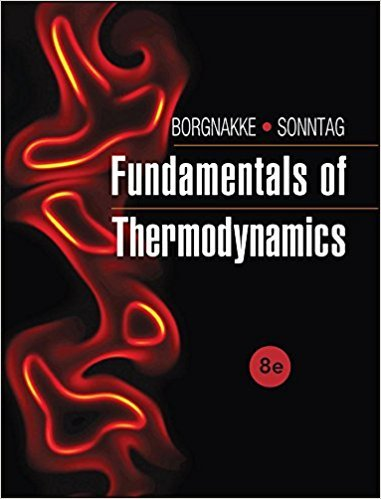×
Get Full Access to Fundamentals Of Thermodynamcs - 8 Edition - Chapter 7 - Problem 113hp
Get Full Access to Fundamentals Of Thermodynamcs - 8 Edition - Chapter 7 - Problem 113hp

×

# Steady Flow ProcessesA coflowing (same direction) heatISBN: 9781118131992 58

## Solution for problem 113HP Chapter 7

Fundamentals of Thermodynamcs | 8th Edition

• Textbook Solutions
• 2901 Step-by-step solutions solved by professors and subject experts
• Get 24/7 help from StudySoup virtual teaching assistantsFundamentals of Thermodynamcs | 8th Edition

4 5 1 392 Reviews
15
3
Problem 113HP

Problem 113HP

A coflowing (same direction) heat exchanger, shown in Fig. P7.113, has one line with 0.5 kg/s oxygen at 17° C, 200 kPa entering, and the other line has 0.6 kg/s nitrogen at 150 kPa, 500 K entering. The heat exchanger is very long, so the two flows exit at the same temperature. Use constant heat capacities and find the exit temperature and the total rate of entropy generation.

FIGURE P7.113Step-by-Step Solution:
Step 1 of 3

Spencer Kociba CHEM 101 Lecture 11/15/16 THERMOCHEMISTRY ● Enthalpy ○ Represented as ΔH ○ Internal energy + pressure*volume ■ We will mostly be concerned with constant pressure. This is where the formula ΔH = ΔE + PΔV comes from ○ ΔH = q (enthalpy=heat gained or lost in the system) ● Properties of Enthalpy (ΔH ) ○ ΔH is directly proportional to mass ○ ΔH for a reverse reaction is equal in magnitude (quantity) but opposite in sign for the forwards reaction ○ Hess’s Law: ΔH is independent of the number of steps involved ■ If Reaction(1) + Reaction(2)=Reaction(3), then ΔH =3ΔH + 1H 2 ● Endothermic reactions ○ Gains heat from sur

Step 2 of 3

Step 3 of 3

##### ISBN: 9781118131992

Since the solution to 113HP from 7 chapter was answered, more than 258 students have viewed the full step-by-step answer. This textbook survival guide was created for the textbook: Fundamentals of Thermodynamcs , edition: 8. Fundamentals of Thermodynamcs was written by and is associated to the ISBN: 9781118131992. This full solution covers the following key subjects: heat, kpa, line, temperature, entering. This expansive textbook survival guide covers 7 chapters, and 1462 solutions. The full step-by-step solution to problem: 113HP from chapter: 7 was answered by , our top Engineering and Tech solution expert on 08/03/17, 05:05AM. The answer to “Steady Flow ProcessesA coflowing (same direction) heat exchanger, shown in Fig. P7.113, has one line with 0.5 kg/s oxygen at 17° C, 200 kPa entering, and the other line has 0.6 kg/s nitrogen at 150 kPa, 500 K entering. The heat exchanger is very long, so the two flows exit at the same temperature. Use constant heat capacities and find the exit temperature and the total rate of entropy generation.FIGURE P7.113” is broken down into a number of easy to follow steps, and 71 words.

Unlock Textbook Solution